Plums

In the bowl are plums.

How many would be there if we can divide it equally among 8, 10 and 11 children?

Result

x =  440

Solution:Leave us a comment of example and its solution (i.e. if it is still somewhat unclear...):Be the first to comment!To solve this example are needed these knowledge from mathematics:

Do you want to calculate least common multiple two or more numbers?

Next similar examples:

1. The port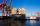Four ships Berthed in the port. Together sail from a port. The first ship will return to port every two weeks, second ship after 4 weeks, third after 8 weeks and fourth ship after 12 weeks. After how many weeks all the boats gather at the port?
2. Matches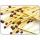George poured out of the box matches and composing them triangles and no match was left. Then he tries squares, hexagons and octagons and no match was left. How many matches must be at least in the box?
3. Digit sumDetermine for how many integers greater than 900 and less than 1,001 has digit sum digit of the digit sum number 1.
4. Divisibility 2How many divisors has integer number 13?
5. BiketrialKamil was biketrial. Before hill he set the forward gear with 42 teeth and the back with 35 teeth. After how many exercises (rotation) of the front wheel both wheels reach the same position?
6. Dance ensemble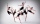The dance ensemble took the stage in pairs. During dancing, the dancers gradually formed groups of four, six and nine. How many dancers have an ensemble?
7. School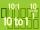Headteacher think whether the distribution of pupils in race in groups of 4,5,6,9 or 10. How many pupils must have at least school at possible options?
8. Cents no more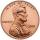Janko bought pencils for 35 cents each. Neither he nor the salesperson had small coins just a whole € 1 coin. At least how many pencils had to buy to pay for the whole euros?
9. Balls groups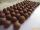Karel pulled the balls out of his pocket and divide it into the groups. He could divide them in four, six or seven, and no ball ever left. How little could be a ball?
10. Two-digit numberI am a two-digit number less than 20. When I divided by three, then you get the rest 1 and when you divide me by four you get also rest 1. What number am I?
11. Three buses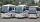Three public transport buses depart together from the bus station in the morning. The first bus was returning to the station after 18 minutes, the second after 12 minutes and a third after 24 minutes. How long will again together on the station? Result exp
12. Game roomWinner can took win in three types of jettons with value 3, 30 and 100 dollars. What is minimal value of win payable in this values of jettons?
13. Counting numberWhat is the smallest counting number divisible by 2,5,7,8 and 15?
14. DivisibilityDetermine all divisors of number 84.
15. Lcm simpleFind least common multiple of this two numbers: 140 175.
16. Write decimalsWrite in the decimal system the short and advanced form of these numbers: a) four thousand seventy-nine b) five hundred and one thousand six hundred and ten c) nine million twenty-six
17. Lcm of three numbersWhat is the Lcm of 120 15 and 5